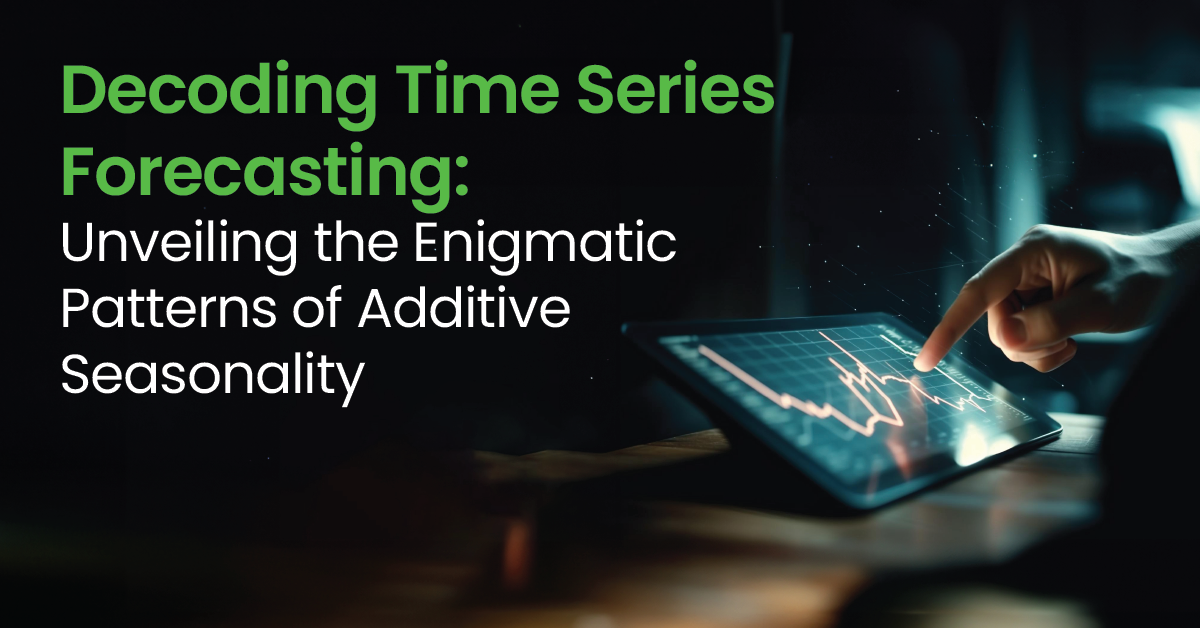Home / Blog / Data Science / What is Poisson Regression? : Flowchart, Models and Analysis

# What is Poisson Regression? : Flowchart, Models and Analysis

• November 03, 2023
• 2312
• 58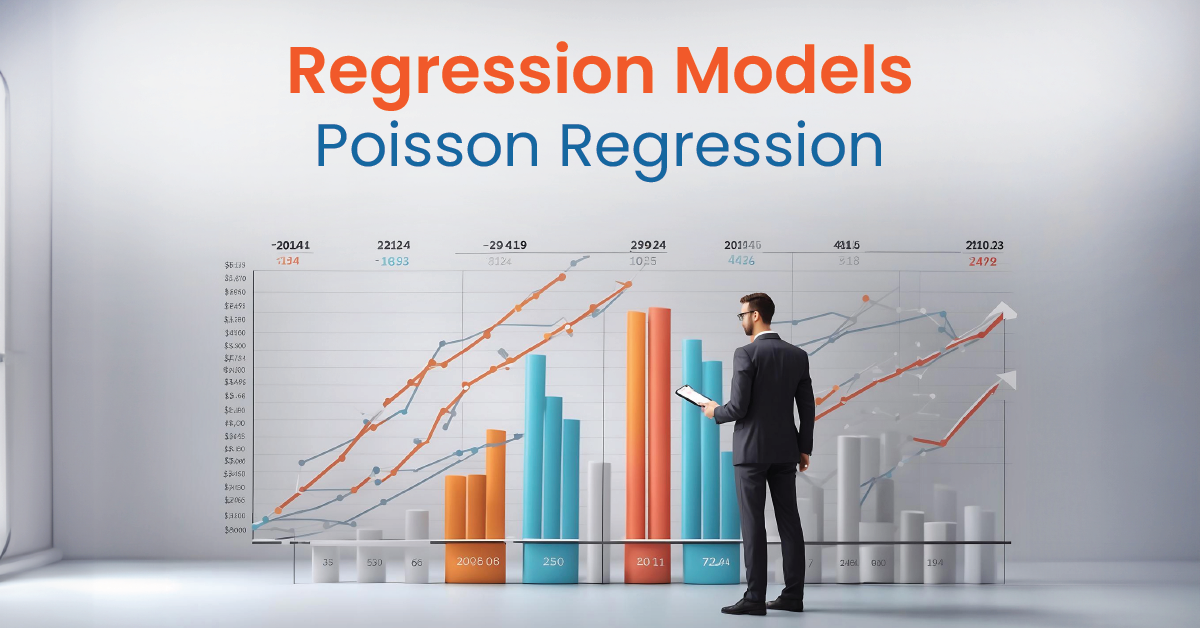### Meet the Author : Mr. Bharani Kumar

Bharani Kumar Depuru is a well known IT personality from Hyderabad. He is the Founder and Director of Innodatatics Pvt Ltd and 360DigiTMG. Bharani Kumar is an IIT and ISB alumni with more than 17 years of experience, he held prominent positions in the IT elites like HSBC, ITC Infotech, Infosys, and Deloitte. He is a prevalent IT consultant specializing in Industrial Revolution 4.0 implementation, Data Analytics practice setup, Artificial Intelligence, Big Data Analytics, Industrial IoT, Business Intelligence and Business Management. Bharani Kumar is also the chief trainer at 360DigiTMG with more than Ten years of experience and has been making the IT transition journey easy for his students. 360DigiTMG is at the forefront of delivering quality education, thereby bridging the gap between academia and industry.

## Introduction

Poisson Regression is a powerful tool in statistics and data science for modelling count data. Poisson Regression is a statistical model used for analysing count data or event data, where the dependent variable represents the number of occurrences of a specific event in a fixed unit of observation.

Unlike linear regression, which is suited for continuous outcomes, Poisson Regression is designed for non-negative integer outcomes, such as the number of customer arrivals, accidents, or product defects. It models the relationship between predictors (independent variables) and the expected count of events, assuming that the event count follows a Poisson distribution.

The model calculates how predictors' effects will affect the event rate while accounting for overdispersion if necessary, making it a valuable tool in various fields like epidemiology, finance, and social sciences. It's particularly useful when you're dealing with data that represents occurrences over a fixed interval, such as website visits, customer arrivals, or disease cases. In this interactive introduction, we'll explore the basics of Poisson Regression, from understanding the Poisson distribution to fitting models and making predictions.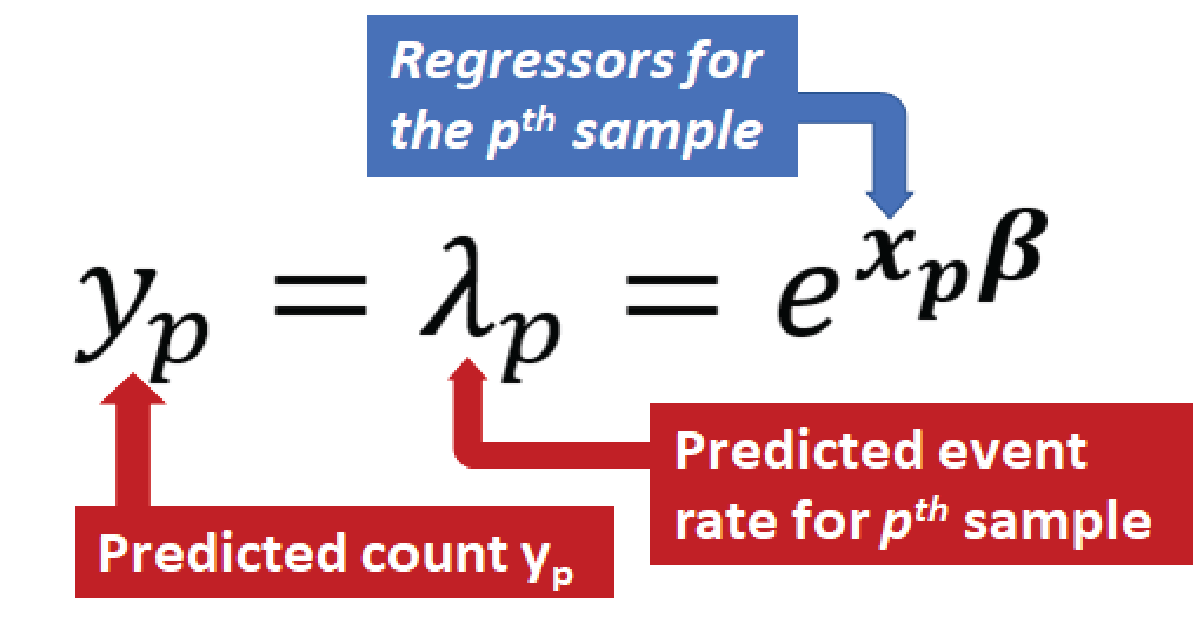Are you looking to become a Data Scientist? Go through 360DigiTMG's Data Science course training in Hyderabad!

## Poisson regression analysis

Key components of Poisson regression analysis include:

1. Independent Variables:These are predictor variables that are used to explain or predict variations in the count of events. Independent variables can be continuous or categorical.

2. Dependent Variable:The quantity is the dependent variable

3. Link Function:Poisson regression employs a logarithmic link function to relate the mean of the Poisson distribution (event rate) to the linear combination of the independent variables. Common link functions include the log and logit links.

4. Model Parameters:In Poisson regression, you estimate model parameters, including coefficients for each independent variable, which indicate the effect of the predictor on the event rate.

5. Overdispersion:Poisson regression assumes that the dependent variable's mean is constant and that its variance is too. However, in some cases, there may be Overdispersion, where the variance is greater than the mean. In such cases, you might consider using a Using a negative binomial regression model, explain this extra variability.

6. Hypothesis Testing:Like other regression models, Poisson regression allows you to perform hypothesis tests to determine if independent variables have a significant impact on the event rate.

## Flowchart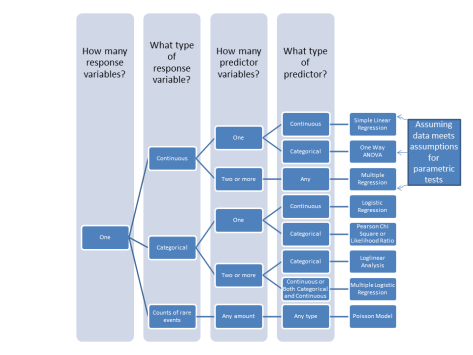## Poisson regression in r

Poisson regression in R can be performed using the glm function (Generalized Linear Models) with a specified family argument set to "poisson." Here's a step-by-step guide on how to perform Poisson regression in R:

2. Fit Poisson Regression Model:Use the glm function to fit a Poisson regression model. Here's the basic syntax:

model <- glm(y ~ x1 + x2 + ..., data = your_data, family = poisson)

• y is your dependent variable (count data).
• x1, x2, ... are your independent variables.
• data is the name of your dataset.
• family is set to "poisson" to specify Poisson regression.

3. Model Summary:You can view the summary statistics of the fitted Poisson regression model using the summary function:

Summary(model)

This will provide information on coefficients, standard errors, p-values, and other relevant statistics.

4. Predictions:You can make predictions using your Poisson regression model for new or existing data points using the predict function:

predictions <- predict (model, newdata = new_data, type = "response")

• new_data is the dataset for which you want to make predictions.
• type = "response" is used to obtain predicted counts.

5. Model Diagnostics:It's important to assess the goodness of fit and check for overdispersion. You can use various diagnostic plots and tests, such as the Deviance Residuals vs. Fitted values plot or the Pearson chi-squared test, to evaluate your model.

6. Interpret Results:Interpret the coefficients and assess the significance of predictor variables in explaining the count data.

Here's a simplified example using a hypothetical dataset: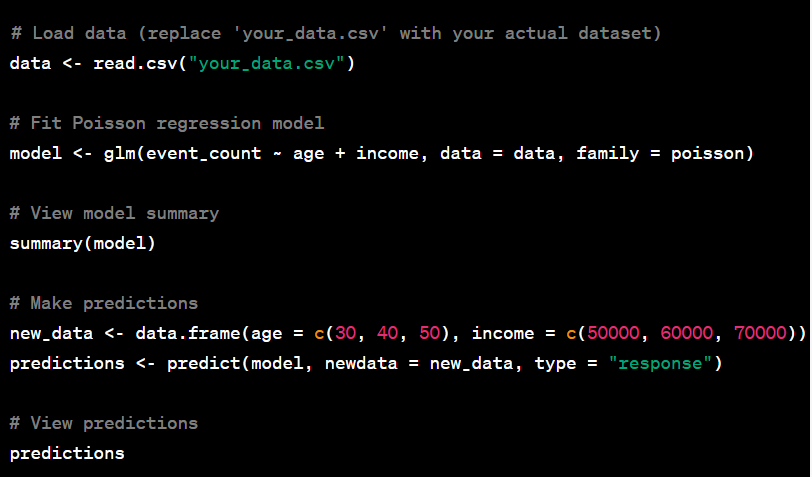This code fits a Poisson regression model, summarizes the results, and makes predictions for new data points. Adjust the variable names and dataset according to your specific analysis.

Want to learn more about data science? Enroll in this Data Science Classes in Bangalore to do so.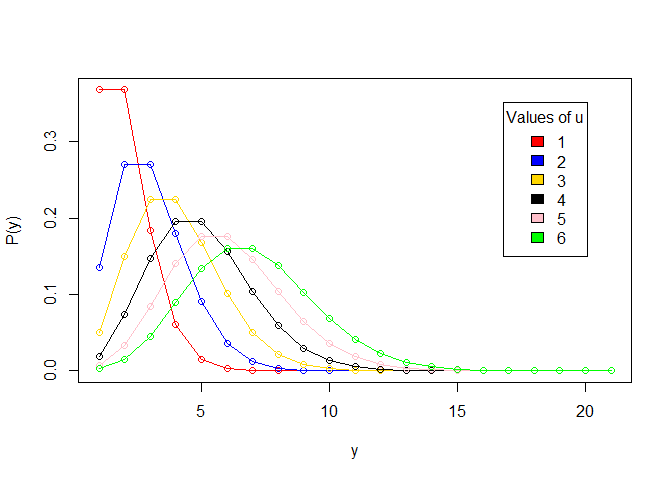## Poisson regression model

A Poisson regression model is a statistical model used to analyze count data or event data. It is specifically designed for situations where the dependent variable represents the number of occurrences of a specific event within a fixed unit of observation. An expansion of the is the Poisson regression model. Poisson distribution, which is characterized by non-negative integer values and often used to describe rare events.

Here are the key components of a Poisson regression model:

1. Dependent Variable: The Y-value of the dependent variable count of events or occurrences. It should be a non-negative integer.

2. Independent Variables: These are predictor variables (X1, X2, X3, etc.) that you believe influence the count of events. Independent variables can be continuous (e.g., temperature) or categorical (e.g., type of product).

3. Link Function:Poisson regression models the relationship between the mean of the Poisson distribution (event rate) and combining the independent variables in a linear fashion. using a logarithmic link function. Common link functions include the log link and the identity link.

3. Link Function:Poisson regression models the relationship between the mean of the Poisson distribution (event rate) and combining the independent variables in a linear fashion. using a logarithmic link function. Common link functions include the log link and the identity link.

4. Model Parameters:The model estimates coefficients (β0, β1, β2, etc.) for each independent variable. These coefficients indicate the effect of the predictors on the expected count of events. The model also estimates the intercept (β0).

5. Assumptions:The Poisson regression model presupposes that the dependant variable's variance equals . This assumption is known as equidispersion. If there is overdispersion (variance > mean), you might consider using a negative binomial regression model instead.

6. Hypothesis Testing:Like other regression models, you can perform hypothesis tests on the coefficients Identify whether the independent variables have an effect on the event rate that is statistically significant.

The following is an example of the Poisson regression model:

log(μ)=β0​+β1​X1​+β2​X2​+…

Where:

log(μ) is the natural logarithm of the expected event rate.

β0​ is the intercept.

β1​,β2​,… are the coefficients for the independent variables.

X1​,X2​,… are the values of the independent variables.

Poisson regression is used in various fields, including epidemiology, finance, social sciences, and more, to model and understand relationships between predictors and event counts. It is a valuable tool for analysing count data and making predictions based on the influence of predictor variables.

## zero inflated poisson regression

Zero-Inflated Poisson Regression (ZIP Regression) is a statistical modelling technique used when dealing with count data that exhibits an excessive number of zero values or "excess zeros."The Poisson regression model is expanded upon in this. that accounts for two types of zeros: structural zeros (zeros that should naturally occur) and excess zeros (zeros that occur more frequently than expected).

## Poisson regression python

Performing Poisson regression in python typically involves using libraries such as statsmodels or scikit-learn.

# Import necessary libraries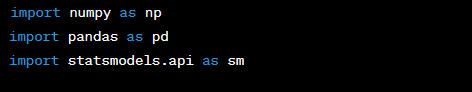# You'll need a dataset with count data as the dependent variable and independent variables.

# For example - simple synthetic dataset: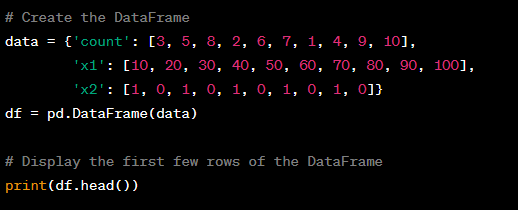# Step 2: Fit Poisson regression model

# Here, we'll fit a Poisson regression model with 'count' as the dependent variable and 'x1' and 'x2' as independent variables.

X = df[['x1', 'x2']] # Independent variables

y = df['count'] # Dependent variable

# Add a constant (intercept) to the model

# Fit the Poisson regression model

poisson_model = sm.GLM(y, X, family=sm.families.Poisson()).fit()

# Step 3: View model summary

print(poisson_model.summary())

# Step 4: Make predictions

# You can make predictions using the fitted model for new data points:

new_data = pd.DataFrame({'x1': [25, 75], 'x2': [1, 0]}) # New data points

predictions = poisson_model.predict(new_data)

print(predictions)

This code snippet demonstrates how to perform Poisson regression in Python using the statsmodels library. Be sure to replace the dataset (df) and independent variables ('x1', 'x2') with your actual data and variables of interest.

Remember to install statsmodels if you haven't already by running pip install statsmodels in your Python environment.

## Poisson regression formula

Poisson regression is a particular sort of generalized linear model (GLM). If the dependent variable is a count, it is appropriate to look at the number of instances of a given event within a set unit of observation.

Typically, the Poisson regression model is written as follows:

log(μ)=β0​+β1​X1​+β2​X2​+…+βp​Xp​

Or, equivalently:

μ=eβ0​+β1​X1​+β2​X2​+…+βp​Xp​SS

360DigiTMG also offers the Data Science Course in Chennai to start a better career. Enroll now!

Poisson regression sas

Performing Poisson regression in SAS (Statistical Analysis System) involves using the GENMOD procedure (Generalized Linear Models) with the appropriate distribution family specified as "Poisson."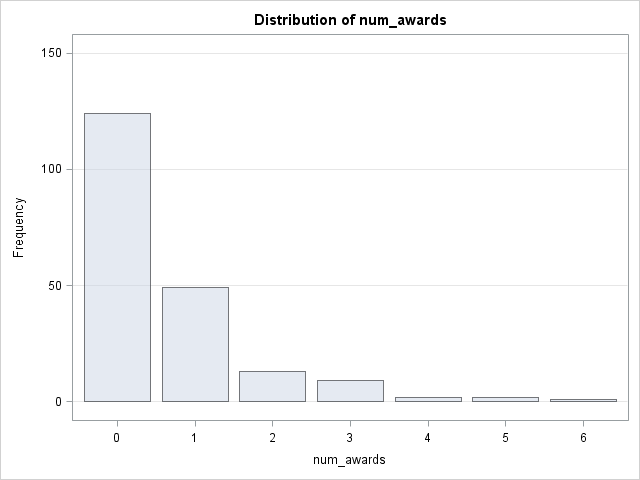## Bayesian poisson regression

Bayesian Poisson regression is a statistical modelling approach that combines Bayesian methods with the Poisson regression model. It allows you to estimate the parameters of a Poisson regression model while incorporating prior information, uncertainty, and probability distributions. This approach is particularly useful when you have limited data and want to make probabilistic inferences about model parameters.

Here's a general overview of how Bayesian Poisson regression works:

1. Model Specification: Define the Poisson regression model, similar to the frequentist approach. The model relates the expected count (rate) of events (μ) to a linear combination of predictor variables (X1​,X2​,…,Xp​) and model coefficients (β0​,β1​,β2​,…,βp​). The key difference is that in Bayesian Poisson regression, the coefficients (βi​) are treated as random variables with probability distributions.

2. Prior Distributions: Specify prior probability distributions for the model coefficients. Priors represent your prior beliefs or information about the coefficients before observing data.

3. Likelihood Function: Define the likelihood function, which describes the probability of observing the data given the model and its parameters. For Poisson regression, the likelihood function follows a Poisson distribution.

4. Bayesian Inference: Use Bayesian methods to update the prior distributions based on the observed data. This involves calculating the posterior distribution of the model parameters using Bayes' theorem.

5. Markov Chain Monte Carlo (MCMC): Bayesian Poisson regression models are often complex, making it challenging to derive analytical solutions for the posterior distribution. Therefore, numerical methods like Markov Chain Monte Carlo (MCMC) are commonly used to sample from the posterior distribution.

Posterior Analysis:strong> Once you have posterior samples, you can conduct various analyses, such as estimating credible intervals for model parameters, making predictions, and assessing model fit. Bayesian Poisson regression provides a full probabilistic framework for inference, which can be valuable for uncertainty quantification.

Popular software packages like Stan, PyMC3, and JAGS can be used to implement Bayesian Poisson regression in practice. These packages simplify the process of specifying models, sampling from posterior distributions, and conducting Bayesian inference.## Generalized poisson regression in r

Generalized Poisson Regression (GPR) is a variation of the Poisson regression model that allows for more flexibility in modelling count data with overdispersion or under dispersion. In GPR, the variance of the response variable is not constrained to be equal to its mean, as it is in traditional Poisson regression. Instead, GPR introduces an additional dispersion parameter to account for overdispersion or under dispersion in the data.

To perform Generalized Poisson Regression in R, you can use the gamlss package, which specializes fitting Location, Scale, and Shape Generalized Additive Models.

## Conclusion

In conclusion, Poisson Regression models offer a valuable tool in statistical analysis when dealing with count data. Unlike traditional linear regression, Poisson Regression is specifically designed for situations where the dependent variable represents non-negative integer values, making it ideal for count-based outcomes.

By accounting for the unique characteristics of count data, such as overdispersion or under dispersion, Poisson Regression provides a robust framework for estimating relationships and making predictions in various fields, including epidemiology, economics, and social sciences. Its flexibility and ability to handle real-world data distributions make it an indispensable tool for researchers seeking to understand and model count-based phenomena accurately.

### Data Analyst Courses in Other Locations